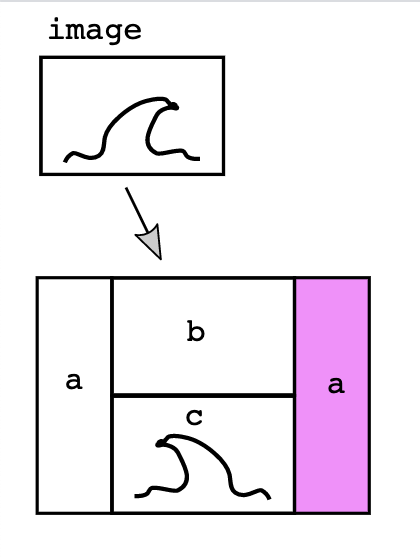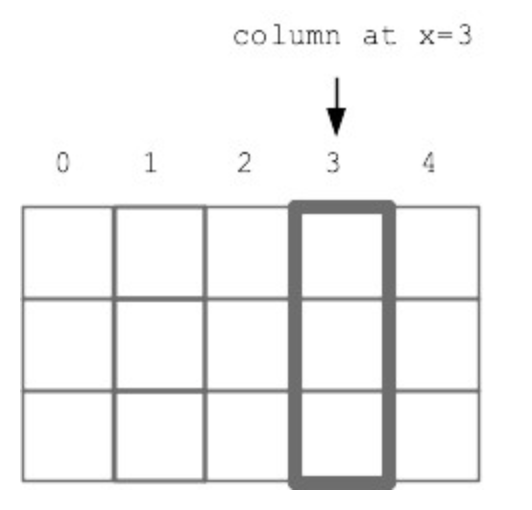# Section #4: Midterm Review

October 17th, 2021

Written by Juliette Woodrow, Brahm Capoor, Nick Parlante, Anna Mistele, and John Dalloul

This week in section you will work through problems that will help you study for the midterm. This handout only has a few problems that we think are great practice problems. Later this week, we will release another handout with more midterm review problems.

## Mid-Quarter Evaluation

Please take the first few minutes of section to fill out the mid-quarter evaluation. Nick and Juliette read each and every piece of feedback that you provide so that we can adjust the course to best meet your needs. Mid-Quarter Evaluation

## Trace Problem

For each ??, write what value comes from the Python expression on the above line.

>>> 1 + 2 - 3 + 4
??
>>>
>>> 3 + 4 * 2
??


## Function Call Tracing

Similar to the above problem, this is a tracing problem. Rather than copying it into Pycharm and running it to see what it prints out, we are going to trace through it by hand to see how values are passed between functions. This may seem like a silly exercise but tracing through code is a good skill to have, especially when you take the midterm as you will not be able to run your code.

        
def main():
x = 4
y = 8
z = x + y
y = b(x, z)
print("Main variables: ", x, y, z)
​
def b(y, x):
z = x + y
print("b variables: ", x, y, z)
if z % 2 == 0: # if (x+y) is even
return z + 1
return z // 2




## Images

#### Wave

Given an image file and int margin that is 0 or more, create and return a new out image as follows (see diagram below). (a) The out image should be created as blank white image as usual, with space for two columns added at its sides, each with the width “margin”. The left column is blank, the right column is colored purple. The purple color can be made with green = 0, red and blue = 255 as on the homework. (b) Between the columns, there should be a top area the size of the original image which is left blank. (c) Below the blank area should be a copy of the image which is reversed horizontally but unchanged vertically.In the diagram, each of the 4 areas is shown with a black border to show where the areas are. There are no black borders in the actual output. The boilerplate code is provided, fill in the missing bits of code:

1. Create the out image
2. for y/x loop code to color in the purple columns
3. for y/x loop code to copy in the reversed image
    
def wave(filename, margin):
image = SimpleImage(filename)

#### Part a Create out ####
pass

#### Part b Color in column ####
pass

#### Part c Copy in reversed ###
pass

return out



## Grid

#### Jump Down

For this problem you will implement a new operation that works on one vertical column of squares in the grid. Every square in the grid is either a rock 'r', sand 's', or empty None. The jumpdown(grid, x, n) algorithm works as follows: The parameter x will be an in-bounds x value, indicating the column to work on. The parameter n will be 1 or more, the number of squares down to move each sand.Look at every "candidate" square in the column, working from the bottom of the column to the top. If the candidate square contains sand, the sand will be moved. Its "destination" square is n squares straight down, (n=1 meaning the square immediately below). If the destination is empty, move the candidate sand there. If the destination is not empty or is out of bounds, then erase the candidate sand from the grid. Obstacles between the candidate sand and its destination do not matter - the sand jumps to its destination.

        
def jumpdown(grid, x, n):
"""
>>> grid = Grid.build([['s', 's'], ['r', None], [None, 'r']])
>>> jumpdown(grid, 0, 2)
[[None, 's'], ['r', None], ['s', 'r']]
>>> grid = Grid.build([['s', 's'], ['r', None], [None, 'r']])
>>> jumpdown(grid, 1, 2)
[['s', None], ['r', None], [None, 'r']]
>>> grid = Grid.build([['s', None], ['s', None], [None, None]])
>>> jumpdown(grid, 0, 1)
[[None, None], ['s', None], ['s', None]]
"""
pass



## Crypto

#### Double Encrypt

This question is from an old quiz.

his problem is similar to the Crypto homework with the simplification that the encryption is done entirely with lowercase chars. This "double" encryption uses two source lists, src1 and src2, and two slug lists, slug1 and slug2. All the lists contain chars and are the same length. Implement a function, encrypt_double(src1, slug1, src2, slug2, char), with the following algorithm: use the lowercase version of the input char, which may be uppercase. If the char is in src1, return the char at the corresponding index in slug1. If the char is in src2, return the char at the corresponding "reverse index" in slug2, e.g. like this for lists length 4:

index     reverse index
0         3
1         2
2         1
3         0


If the char is not in src1 or src2, return the char unchanged. No char appears in both src1 and src2.

Double Encrypt Example (len 4)
src1  = ['a', 'b', 'c', 'd']
slug1 = ['s', 't', 'u', 'v']
src2  = ['e', 'f', 'g', 'h']
slug2 = ['w', 'x', 'y', 'z']
encrypt 'A' -> 's'
encrypt 'e' -> 'z'
encrypt 'f' -> 'y'
encrypt '$' -> '$'


## String Parsing

#### Product in Brackets

Implement a function, prod_in_brackets(s), which takes in a string s. The string s will be in the form 'xxx y  cs python{6}zz' where there are two types of brackets (in this example there are square brackets [] and curly brackets {}). If there are brackets present, there will be numbers inside of them. The function should return the product of the numbers inside the two brackets.

You can assume that if present, the curly brackets will always be after the square brackets. You can also assume that if there is a left bracket there will be a right bracket of the same type following it and that the strings inside the brackets can be converted to integers. If there are only square brackets, return the number inside those brackets. If there are no brackets at all, return None.

For example,
>>> s = 'xxx y  cs python{6}zz'
>>> prod_in_brackets(s)
60
>>> s = 'zzyctr'
>>> prod_in_brackets(s)
55
>>> s = 'CS106A'
>>> prod_in_brackets(s)
>>>